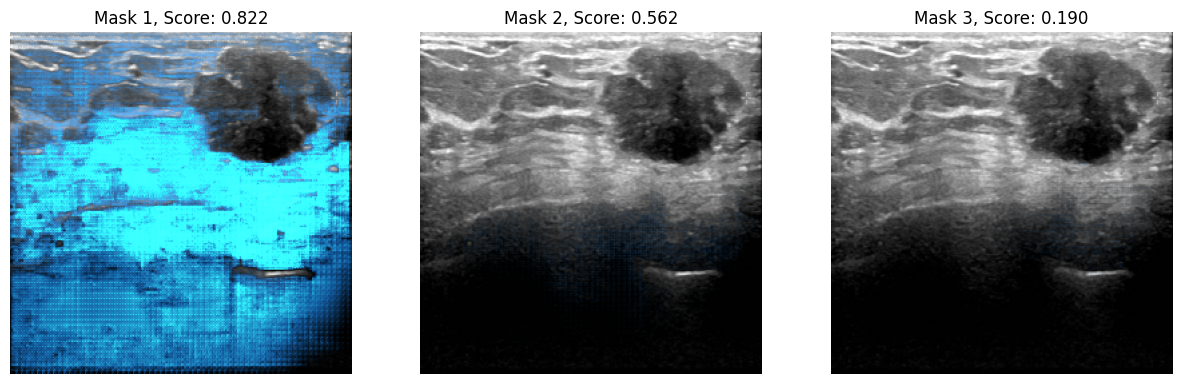Code examples / Computer Vision / Segment Anything Model with 🤗Transformers

# Segment Anything Model with 🤗Transformers

Authors: Merve Noyan & Sayak Paul
Date created: 2023/07/11
Description: Fine-tuning Segment Anything Model using Keras and 🤗 Transformers.

ⓘ This example uses Keras 2View in ColabGitHub source

## Introduction

Large language models (LLMs) make it easy for the end users to apply them to various applications through "prompting". For example if we wanted an LLM to predict the sentiment of the following sentence — "That movie was amazing, I thoroughly enjoyed it" — we'd do prompt the LLM with something like:

What's the sentiment of the following sentence: "That movie was amazing, I thoroughly enjoyed it"?

In return, the LLM would return sentiment token.

But when it comes to visual recognition tasks, how can we engineer "visual" cues to prompt foundation vision models? For example, we could have an input image and prompt the model with bounding box on that image and ask it to perform segmentation. The bounding box would serve as our visual prompt here.

In the Segment Anything Model (dubbed as SAM), researchers from Meta extended the space of language prompting to visual prompting. SAM is capable of performing zero-shot segmentation with a prompt input, inspired by large language models. The prompt here can be a set of foreground/background points, free text, a box or a mask. There are many downstream segmentation tasks, including semantic segmentation and edge detection. The goal of SAM is to enable all of these downstream segmentation tasks through prompting.

In this example, we'll learn how to use the SAM model from 🤗 Transformers for performing inference and fine-tuning.

## Installation

``````!!pip install -q git+https://github.com/huggingface/transformers
``````
``````[]
``````

Let's import everything we need for this example.

``````from tensorflow import keras
from transformers import TFSamModel, SamProcessor
import numpy as np
import tensorflow as tf
import matplotlib.pyplot as plt
from tensorflow.python.ops.numpy_ops import np_config
from PIL import Image
import requests
import glob
import os
``````
``````/Users/mervenoyan/miniforge3/envs/py310/lib/python3.10/site-packages/tqdm/auto.py:21: TqdmWarning: IProgress not found. Please update jupyter and ipywidgets. See https://ipywidgets.readthedocs.io/en/stable/user_install.html
from .autonotebook import tqdm as notebook_tqdm
``````

## SAM in a few words

SAM has the following components:

||:—:| | Image taken from the official SAM blog post | |

The image encoder is responsible for computing image embeddings. When interacting with SAM, we compute the image embedding one time (as the image encoder is heavy) and then reuse it with different prompts mentioned above (points, bounding boxes, masks).

Points and boxes (so-called sparse prompts) go through a lightweight prompt encoder, while masks (dense prompts) go through a convolutional layer. We couple the image embedding extracted from the image encoder and the prompt embedding and both go to a lightweight mask decoder. The decoder is responsible for predicting the mask.Figure taken from the SAM paper

SAM was pre-trained to predict a valid mask for any acceptable prompt. This requirement allows SAM to output a valid mask even when the prompt is ambiguous to understand — this makes SAM ambiguity-aware. Moreover, SAM predicts multiple masks for a single prompt.

We highly encourage you to check out the SAM paper and the blog post to learn more about the additional details of SAM and the dataset used to pre-trained it.

## Running inference with SAM

There are three checkpoints for SAM:

We load `sam-vit-base` in `TFSamModel`. We also need `SamProcessor`for the associated checkpoint.

``````model = TFSamModel.from_pretrained("facebook/sam-vit-base")
``````
``````All model checkpoint layers were used when initializing TFSamModel.
``````
``````All the layers of TFSamModel were initialized from the model checkpoint at facebook/sam-vit-base.
If your task is similar to the task the model of the checkpoint was trained on, you can already use TFSamModel for predictions without further training.
``````

Next, we write some utility functions for visualization. Most of these functions are taken from this notebook.

``````np_config.enable_numpy_behavior()

if random_color:
color = np.concatenate([np.random.random(3), np.array([0.6])], axis=0)
else:
color = np.array([30 / 255, 144 / 255, 255 / 255, 0.6])

def show_box(box, ax):
x0, y0 = box, box
w, h = box - box, box - box
plt.Rectangle((x0, y0), w, h, edgecolor="green", facecolor=(0, 0, 0, 0), lw=2)
)

def show_boxes_on_image(raw_image, boxes):
plt.figure(figsize=(10, 10))
plt.imshow(raw_image)
for box in boxes:
show_box(box, plt.gca())
plt.axis("on")
plt.show()

def show_points_on_image(raw_image, input_points, input_labels=None):
plt.figure(figsize=(10, 10))
plt.imshow(raw_image)
input_points = np.array(input_points)
if input_labels is None:
labels = np.ones_like(input_points[:, 0])
else:
labels = np.array(input_labels)
show_points(input_points, labels, plt.gca())
plt.axis("on")
plt.show()

def show_points_and_boxes_on_image(raw_image, boxes, input_points, input_labels=None):
plt.figure(figsize=(10, 10))
plt.imshow(raw_image)
input_points = np.array(input_points)
if input_labels is None:
labels = np.ones_like(input_points[:, 0])
else:
labels = np.array(input_labels)
show_points(input_points, labels, plt.gca())
for box in boxes:
show_box(box, plt.gca())
plt.axis("on")
plt.show()

def show_points_and_boxes_on_image(raw_image, boxes, input_points, input_labels=None):
plt.figure(figsize=(10, 10))
plt.imshow(raw_image)
input_points = np.array(input_points)
if input_labels is None:
labels = np.ones_like(input_points[:, 0])
else:
labels = np.array(input_labels)
show_points(input_points, labels, plt.gca())
for box in boxes:
show_box(box, plt.gca())
plt.axis("on")
plt.show()

def show_points(coords, labels, ax, marker_size=375):
pos_points = coords[labels == 1]
neg_points = coords[labels == 0]
ax.scatter(
pos_points[:, 0],
pos_points[:, 1],
color="green",
marker="*",
s=marker_size,
edgecolor="white",
linewidth=1.25,
)
ax.scatter(
neg_points[:, 0],
neg_points[:, 1],
color="red",
marker="*",
s=marker_size,
edgecolor="white",
linewidth=1.25,
)

if scores.shape == 1:
final_scores = tf.squeeze(scores)

nb_predictions = scores.shape[-1]
fig, axes = plt.subplots(1, nb_predictions, figsize=(15, 15))

axes[i].imshow(np.array(raw_image))
axes[i].axis("off")
plt.show()
``````

We will segment a car image using a point prompt. Make sure to set `return_tensors` to `tf` when calling the processor.

Let's load an image of a car and segment it.

``````img_url = "https://huggingface.co/ybelkada/segment-anything/resolve/main/assets/car.png"
raw_image = Image.open(requests.get(img_url, stream=True).raw).convert("RGB")
plt.imshow(raw_image)
plt.show()
``````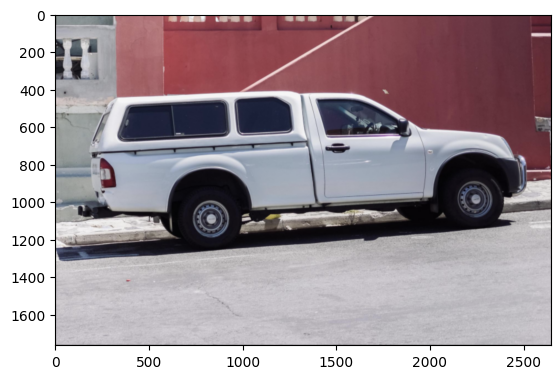Let's now define a set of points we will use as the prompt.

``````input_points = [[[450, 600]]]

# Visualize a single point.
show_points_on_image(raw_image, input_points)
``````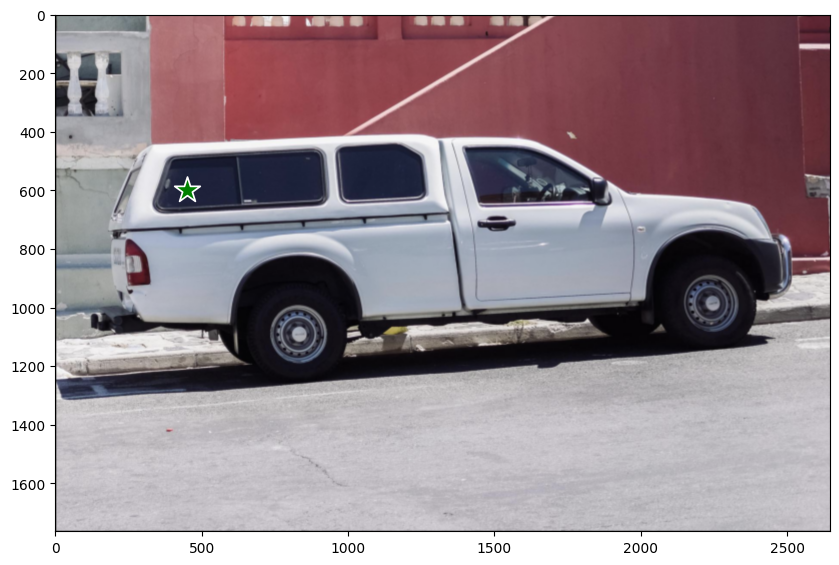And segment:

``````# Preprocess the input image.
inputs = processor(raw_image, input_points=input_points, return_tensors="tf")

# Predict for segmentation with the prompt.
outputs = model(**inputs)
``````

`outputs` has got two attributes of our interest:

• `outputs.pred_masks`: which denotes the predicted masks.
• `outputs.iou_scores`: which denotes the IoU scores associated with the masks.

Let's post-process the masks and visualize them with their IoU scores:

``````masks = processor.image_processor.post_process_masks(
inputs["original_sizes"],
inputs["reshaped_input_sizes"],
return_tensors="tf",
)

``````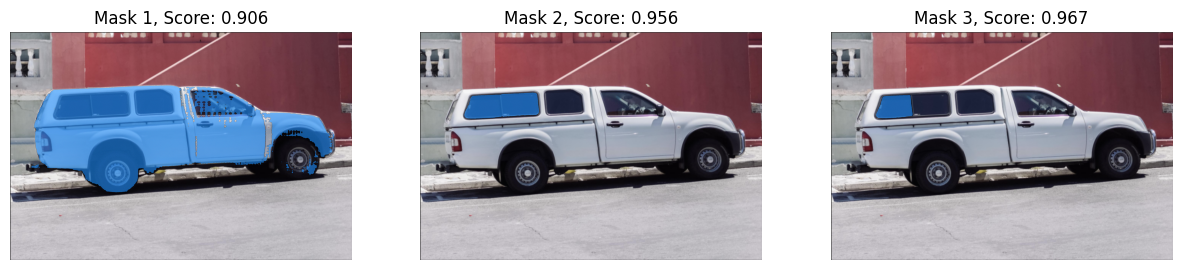And there we go!

As can be noticed, all the masks are valid masks for the point prompt we provided.

SAM is flexible enough to support different visual prompts and we encourage you to check out this notebook to know more about them!

## Fine-tuning

We'll use this dataset consisting of breast cancer scans. In the medical imaging domain, being able to segment the cells containing malignancy is an important task.

### Data preparation

Let's first get the dataset.

``````remote_path = "https://hf.co/datasets/sayakpaul/sample-datasets/resolve/main/breast-cancer-dataset.tar.gz"
dataset_path = keras.utils.get_file(
"breast-cancer-dataset.tar.gz", remote_path, untar=True
)
``````

Let's now visualize a sample from the dataset.

(The `show_mask()` utility is taken from this notebook)

``````def show_mask(mask, ax, random_color=False):
if random_color:
color = np.concatenate([np.random.random(3), np.array([0.6])], axis=0)
else:
color = np.array([30 / 255, 144 / 255, 255 / 255, 0.6])

# Load all the image and label paths.
image_paths = sorted(glob.glob(os.path.join(dataset_path, "images/*.png")))
label_paths = sorted(glob.glob(os.path.join(dataset_path, "labels/*.png")))

# Load the image and label.
idx = 15
image = Image.open(image_paths[idx])
label = Image.open(label_paths[idx])
image = np.array(image)
ground_truth_seg = np.array(label)

# Display.
fig, axes = plt.subplots()
axes.imshow(image)
axes.axis("off")
plt.show()

tf.shape(ground_truth_seg)
``````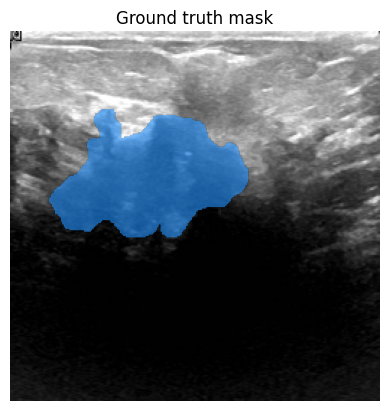``````<tf.Tensor: shape=(2,), dtype=int32, numpy=array([256, 256], dtype=int32)>
``````

### Preparing `tf.data.Dataset`

We now write a generator class to prepare the images and the segmentation masks using the `processor` utilized above. We will leverage this generator class to create a `tf.data.Dataset` object for our training set by using `tf.data.Dataset.from_generator()`. Utilities of this class have been adapted from this notebook.

The generator is responsible for yielding the preprocessed images and the segmentation masks, and some other metadata needed by the SAM model.

``````class Generator:
"""Generator class for processing the images and the masks for SAM fine-tuning."""

def __init__(self, dataset_path, processor):
self.dataset_path = dataset_path
self.image_paths = sorted(
glob.glob(os.path.join(self.dataset_path, "images/*.png"))
)
self.label_paths = sorted(
glob.glob(os.path.join(self.dataset_path, "labels/*.png"))
)
self.processor = processor

def __call__(self):
for image_path, label_path in zip(self.image_paths, self.label_paths):
image = np.array(Image.open(image_path))

# get bounding box prompt

# prepare image and prompt for the model
inputs = self.processor(image, input_boxes=[[prompt]], return_tensors="np")

# remove batch dimension which the processor adds by default
inputs = {k: v.squeeze(0) for k, v in inputs.items()}

yield inputs

def get_bounding_box(self, ground_truth_map):
# get bounding box from mask
y_indices, x_indices = np.where(ground_truth_map > 0)
x_min, x_max = np.min(x_indices), np.max(x_indices)
y_min, y_max = np.min(y_indices), np.max(y_indices)

# add perturbation to bounding box coordinates
H, W = ground_truth_map.shape
x_min = max(0, x_min - np.random.randint(0, 20))
x_max = min(W, x_max + np.random.randint(0, 20))
y_min = max(0, y_min - np.random.randint(0, 20))
y_max = min(H, y_max + np.random.randint(0, 20))
bbox = [x_min, y_min, x_max, y_max]

return bbox
``````

`get_bounding_box()` is responsible for turning the ground-truth segmentation maps into bounding boxes. These bounding boxes are fed to SAM as prompts (along with the original images) during fine-tuning and SAM is then trained to predict valid masks.

The advantage of this first creating a generator and then using it to create a `tf.data.Dataset` is the flexbility. Sometimes, we may need to use utitlities from other libraries (`albumentations`, for example) which may not come in native TensorFlow implementations. By using this workflow, we can easily accommodate such use case.

But the non-TF counterparts might introduce performance bottlenecks, though. However, for our example, it should work just fine.

Now, we prepare the `tf.data.Dataset` from our training set.

``````# Define the output signature of the generator class.
output_signature = {
"pixel_values": tf.TensorSpec(shape=(3, None, None), dtype=tf.float32),
"original_sizes": tf.TensorSpec(shape=(None,), dtype=tf.int64),
"reshaped_input_sizes": tf.TensorSpec(shape=(None,), dtype=tf.int64),
"input_boxes": tf.TensorSpec(shape=(None, None), dtype=tf.float64),
}

# Prepare the dataset object.
train_dataset_gen = Generator(dataset_path, processor)
train_ds = tf.data.Dataset.from_generator(
train_dataset_gen, output_signature=output_signature
)
``````

Next, we configure the dataset for performance.

``````auto = tf.data.AUTOTUNE
batch_size = 2
shuffle_buffer = 4

train_ds = (
train_ds.cache()
.shuffle(shuffle_buffer)
.batch(batch_size)
.prefetch(buffer_size=auto)
)
``````

Take a single batch of data and inspect the shapes of the elements present inside of it.

``````sample = next(iter(train_ds))
for k in sample:
print(k, sample[k].shape, sample[k].dtype, isinstance(sample[k], tf.Tensor))
``````
``````pixel_values (2, 3, 1024, 1024) <dtype: 'float32'> True
original_sizes (2, 2) <dtype: 'int64'> True
reshaped_input_sizes (2, 2) <dtype: 'int64'> True
input_boxes (2, 1, 4) <dtype: 'float64'> True
ground_truth_mask (2, 256, 256) <dtype: 'int32'> True
``````

### Training

We will now write DICE loss. This implementation is based on MONAI DICE loss.

``````def dice_loss(y_true, y_pred, smooth=1e-5):
y_pred = tf.sigmoid(y_pred)
reduce_axis = list(range(2, len(y_pred.shape)))
if batch_size > 1:
# reducing spatial dimensions and batch
reduce_axis =  + reduce_axis
intersection = tf.reduce_sum(y_true * y_pred, axis=reduce_axis)
y_true_sq = tf.math.pow(y_true, 2)
y_pred_sq = tf.math.pow(y_pred, 2)

ground_o = tf.reduce_sum(y_true_sq, axis=reduce_axis)
pred_o = tf.reduce_sum(y_pred_sq, axis=reduce_axis)
denominator = ground_o + pred_o
# calculate DICE coefficient
loss = 1.0 - (2.0 * intersection + 1e-5) / (denominator + 1e-5)
loss = tf.reduce_mean(loss)

return loss
``````

## Fine-tuning SAM

We will now fine-tune SAM's decoder part. We will freeze the vision encoder and prompt encoder layers.

``````# initialize SAM model and optimizer

for layer in sam.layers:
if layer.name in ["vision_encoder", "prompt_encoder"]:
layer.trainable = False

@tf.function
def train_step(inputs):
# pass inputs to SAM model
outputs = sam(
pixel_values=inputs["pixel_values"],
input_boxes=inputs["input_boxes"],
training=True,
)

# calculate loss over predicted and ground truth masks
# update trainable variables
trainable_vars = sam.trainable_variables

return loss
``````
``````All model checkpoint layers were used when initializing TFSamModel.
``````
``````All the layers of TFSamModel were initialized from the model checkpoint at facebook/sam-vit-base.
If your task is similar to the task the model of the checkpoint was trained on, you can already use TFSamModel for predictions without further training.
``````

We can now run the training for three epochs. We might have a warning about gradients not existing on IoU prediction head of mask decoder, we can safely ignore that.

``````# run training
for epoch in range(3):
for inputs in train_ds:
loss = train_step(inputs)
print(f"Epoch {epoch + 1}: Loss = {loss}")
``````
``````WARNING:tensorflow:Gradients do not exist for variables ['tf_sam_model_1/mask_decoder/iou_prediction_head/proj_in/kernel:0', 'tf_sam_model_1/mask_decoder/iou_prediction_head/proj_in/bias:0', 'tf_sam_model_1/mask_decoder/iou_prediction_head/proj_out/kernel:0', 'tf_sam_model_1/mask_decoder/iou_prediction_head/proj_out/bias:0', 'tf_sam_model_1/mask_decoder/iou_prediction_head/layers_._0/kernel:0', 'tf_sam_model_1/mask_decoder/iou_prediction_head/layers_._0/bias:0'] when minimizing the loss. If you're using `model.compile()`, did you forget to provide a `loss` argument?

Epoch 1: Loss = 0.08322787284851074
Epoch 2: Loss = 0.05677264928817749
Epoch 3: Loss = 0.07764029502868652
``````

### Serialize the model

We serialized the model and pushed for you below. `push_to_hub` method serializes model, generates a model card and pushes it to Hugging Face Hub, so that other people can load the model using `from_pretrained` method to infer or further fine-tune. We also need to push the same preprocessor in the repository. Find the model and the preprocessor here.

``````# sam.push_to_hub("merve/sam-finetuned")
# processor.push_to_hub("merve/sam-finetuned")
``````

We can now infer with the model.

``````# Load another image for inference.
idx = 20
raw_image_inference = Image.open(image_paths[idx])

# process the image and infer
preprocessed_img = processor(raw_image_inference)
outputs = sam(preprocessed_img)
``````

Lastly, we can visualize the results.

``````infer_masks = outputs["pred_masks"]
iou_scores = outputs["iou_scores"]
``````WARNING:matplotlib.image:Clipping input data to the valid range for imshow with RGB data ([0..1] for floats or [0..255] for integers).